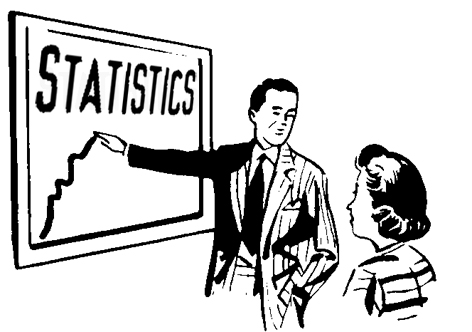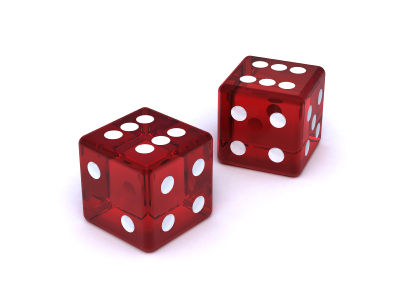# Difference between Probability and Statistics

Statistics is a scientific field that studies the changes of data on mass phenomena and processes over time. It includes methods for the collection, storage and processing of data, analysis and interpretation. It is an indispensable tool of planning and management, which enable you to get the most objective assessment and make predictions as close to reality.

Probability, on the other hand, is used for a probability of an event, In order to calculate the probability, you need to apply the basic concepts of the theory, count the number of all possible events, to get the most accurate result.

### Instructions

• 1

Statistics

Statistical data are stored and processed. With advances in technology there is no problem to use the information in its entirety and process. The existing mathematical theory of statistical measurements allows obtaining the most reliable results that exclude the factors that have an impact on the random processes. This theory allows the development of indicators that best reflect the real processes.

Mathematical statistics is used in many fields of engineering and the hard sciences, to describe natural processes in nature and society. Problem of mathematical statistics is the development of methods for data processing and analysis, checking their validity, terms of use and evaluation of resistance to change these conditions. The tasks to be solved by applied statistics include description, working principles of generalization and explanation of the phenomena of nature to the development of methods to predict or explain these phenomena.

The use of statistical methods for data processing allows a high proportion of the correctness and accuracy of the results, sampling and predict them for the entire population.

Through statistical analysis, we can obtain objective assessments of almost all scientific phenomena and those that characterize the life of society. The interpretation of statistical indicators helps to make science-based management decisions.

Image courtesy: xda-developers.com• 2

Probability

Probability is called the ratio of the total number of favourable outcomes to the total number of tests. Probability of an event is, in fact, the proportion of confidence that a particular result will come or not. Tossing a coin is the simplest example of determining probability.

To determine the conditional probability there are several theories and methods. One way is the multiplication theorem of probability. It explains the probability of a product of events, i.e. the possibility of co-occurrence of these events is the product of the probability of one of these events, the conditional probability for another event, calculated under the condition that the first event has occurred.

Image courtesy: questgarden.com•